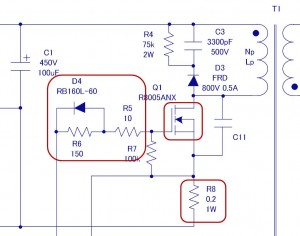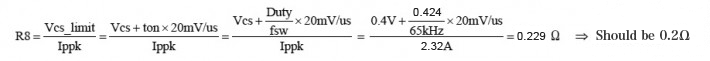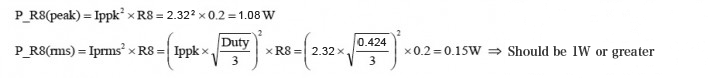Technical Information Site of Power Supply Design

• Basic Knowledge
• AC/DC
• Design Method of PWM AC/DC Flyback Converters
• Designing Isolated Flyback Converter Circuits: Selecting Critical Components – MOSFET related - 2

2016.06.23 AC/DC

# Designing Isolated Flyback Converter Circuits: Selecting Critical Components – MOSFET related - 2

Design Method of PWM AC/DC Flyback Converters

Having finished the selection of MOSFET Q1 in the section [Selecting Critical Components – MOSFET related - 1], we now move on to the step of configuring a circuit around the MOSFET.

First, let us review the operation of the circuit. The signal from the OUT (PWM output) pin from the IC is adjusted in the D4, R5, and R6 to ensure that the MOSFET Q1 operates properly, and to drive the gate of the MOSFET. The MOSFET Q1 turns the rectified high voltage on and off that was input to the primary side of the transformer T1, and transmits the energy of the high voltage to the secondary side. When Q1 turns on, Ids flows. However, since the current cannot be allowed to flow indefinitely, the R8 is used to detect and limit the current.

In this section, we first determine the circuit (diode D4, resistors R5 and R6) that adjusts the gate drive for the MOSFET. Subsequently, we also determine the current sense resistor R8 that is necessary for current sense and slope compensation.

MOSFET gate circuit, R5, R6, and D4In order to drive the MOSFET, signal is output from the PWM output pin of the power supply IC. Since an optimal operation cannot be ensured by simply connecting the signal to the gate of the MOSFET, adjustments are needed to be consistent with the circuit and required properties. In concrete terms, the MOSFET’s switching loss and noise must be optimized.

We adjust the on- and off-speeds (rise and fall time) of the MOSFET so that the MOSFET operates in a manner that represents a compromise between switching loss and switching noise. A compromise is needed because switching loss and switching noise are mutually conflicting factors: Increasing the switching speed reduces the switching loss, whereas the greater the switching speed, creating sharp current changes, the greater is the resulting switching noise.

It is difficult to calculate gate circuit constants based on fixed formulas. Therefore, beginning with the values that are shown in the circuit diagrams on the power supply IC datasheet, finally operate them on an actual device to determine whether the rise in MOSFET temperature is within the allowable range, that is, to evaluate the extent of switching loss. In addition, you need to measure the switching noise to see if it is in a proper range.

• When the MOSFET is on, adjust the speed with R5 and R6
• When the MOSFET is off, adjust the speed with R5 through the fast gate discharge diode D4

In the discontinuous mode for the current mode, which represents a selected operation, basically no switching loss occurs when the MOSFET is on, and the loss occurring during off-time becomes predominant. To reduce switching loss when the MOSFET is off, the speed must be increased by reducing the R5; this approach, however, causes sharp current changes, resulting in an increased switching noise. In the example circuit provided in this section, the following data emerge:

• R5＝22Ω 0.25W、R6＝150Ω、D4：RB160L-60 (Schottky diode 60V/1A)

The diode D4 is used to rapidly discharge the gate charges when the MOSFET is off. A Schottky barrier diode is selected for this purpose by virtue of its low loss and high-speed operation.

As a precaution, since a pulse current flows into the R5, the pulse tolerance of the resistor to be used should be verified.

Current sense resistor R8

The current sense resistor R8 is connected to the source of the MOSFET. One end (for the source) is connected to the CS pin of the power supply IC, and the other end is connected to GND. The CS pin functions through the use of a voltage drop that is generated by the current flowing to the R8 when the MOSFET is on. The resistor performs three functions: limiting the current flowing to the primary side, providing protection against an output overload, and determining slope compensation through the current mode control. For further details on the CS pin, see the Power Supply IC BM1P061FJ Datasheet.

Because it performs multiple functions, the resistor may be subject to restrictions by the primary side inductance of the transformer and by the input voltage. For this reason, the resistor R8 is calculated according to the formula given below, where the values of Ippk and Duty are determined according to [Transformer Design (Calculating numerical values)]. Based on the CS pin voltage standard for BM1P061FJ, the value of Vcs turns out to be 0.4V.The calculation yields an R8 value of 0.2Ω.

Also, the P_R8 value, which represents the loss due to the sense resistor R8, can be determined according to the following equations:By taking into consideration the results of the calculations and the pulse tolerance, the value 1W or greater is identified to be an allowable resistor. With regard to pulse tolerance, because for a given power rating the pulse tolerance changes by the structure of the resistor, it should be checked with the manufacturer of the resistor to be used.

Thus, we have determined the values for the components around the MOSFET. Although the approach, requiring empirical evaluations and verifications by means of an actual device and involving more than formula calculations, may lack in clarity, remember that such is not uncommon in the power supply design process.

#### Key Points:

・The circuits for the control of switching transistors (MOSFET) operation are based on the specifications of the power supply IC.

・Power supply IC datasheets provide circuits and component values determination methods; such information should be consulted in designing a circuit.